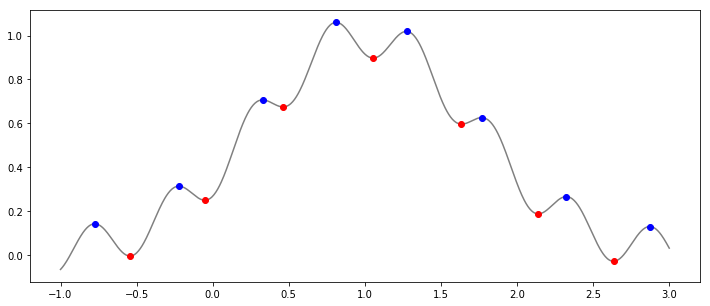#### Numpy: find peaks and valleys¶

When the graph is not too noisy we can use following snippet where numpy detects the local minimums and local maximums of the function. In case of noisy data we would need to smooth out the data for example with polynomial fit.

In :
import numpy as np
import matplotlib.pyplot as plt
%matplotlib inline

# example data with peaks:
x = np.linspace(-1,3,1000)
data = -0.1*np.cos(12*x)+ np.exp(-(1-x)**2)

#     ___ detection of local minimums and maximums ___

a = np.diff(np.sign(np.diff(data))).nonzero() + 1               # local min & max
b = (np.diff(np.sign(np.diff(data))) > 0).nonzero() + 1         # local min
c = (np.diff(np.sign(np.diff(data))) < 0).nonzero() + 1         # local max
# +1 due to the fact that diff reduces the original index number

# plot
plt.figure(figsize=(12, 5))
plt.plot(x, data, color='grey')
plt.plot(x[b], data[b], "o", label="min", color='r')
plt.plot(x[c], data[c], "o", label="max", color='b')
plt.show()Given the ability to detect peaks and valleys in smoothed dataset we can build relatively simple, yet powerful stock screener that would be looking for divergence between stock price and corresponding RSI values.